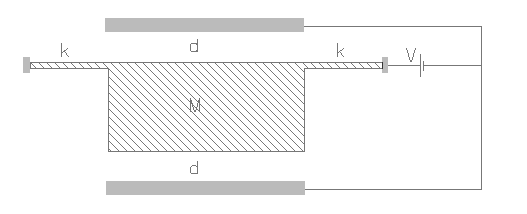ACLSTEP,k,area,m,gap,volt,np,rper,sel
Capacitive accelerometer for sensing a step signal

k            spring constant of the beam in N/m
area      overlapping area of the electrodes in µm2
m           inertial mass in kg
gap        distance between the stationary and movable plate in µm
volt        applied voltage in Volt
np          number of active stationary plates, 1 plate by default
rper       relative permittivity of the medium, it is approximately 1 for air
sel          number denoting the selected result.
Use 1 for maximum acceleration and 3 for maximum displacementNotes

This interface could be used for designing a capacitive accelerometer meant to sense a step signal. Step signal is of more practical significance to an accelerometer design as the accelerometer should be designed keeping in mind any shock loads that it may have to endure. In the case of a step signal, the acceleration magnitude will be zero at time t=0 which then instantaneously reaches the steady state acceleration level. Due to this, the seismic mass will move farther than that in the case of static acceleration and would thereby reduce the range of operation of the accelerometer.

The accelerometer has a mass suspended from a beam with a known spring constant. The stationary electrode can be one or two as shown in the figure above. For capacitance measurement an electrical excitation is applied between the stationary and movable electrodes. This electrical excitation also exerts an electrostatic attraction on the mass which has the movable electrode. The combination of the electrostatic force and the inertial force should not be large enough to send the mass into a pulled-in state where the mass hits one of the stationary electrodes. For single sided excitation, the mass has an offset from the equilibrium position due to the electrical excitation. For double sided excitation, this effect is cancelled off.

This design form can be used to determine the maximum acceleration the device will be able to sense without going into a pull-in state. It also predicts the maximum displacement of the mass. The capacitor design or the applied voltage can be changed to increase or decrease the range of operation. The applied voltage usually has a AC component and a DC component typically in the form of ±V1±V2sinωt where frequency ω is much larger than the frequency of the signal and the natural frequency of vibration of the accelerometer. The effective applied voltage can be expressed as

V = √(V12 + V22∕2)

The plot shows applied acceleration measured in terms of acceleration due to gravity g versus the displacement of the movable electrode for both single sided and double sided excitation. The plot shows that for a given capacitor design, excitation voltage and acceleration load, there is only one stable solution for displacement. The peak point in the curve is the maximum acceleration beyond which there is no stable solution. The corresponding displacement is the maximum stable displacement. If an acceleration above the maximum limit is applied it will go into a pulled-in state. It can also be seen that for double sided excitation, the operational range is larger than for single sided excitation. Larger reliable operational range would mean a lower sensitivity and hence is a trade-off.

Assumptions

-Step acceleration signal normal to the plane of the mass is assumed.
-Damping effects are not considered.
-The stationary electrodes are arranged symmetrically on both sides of the movable electrode.
-The spring constant of the beam is known. If unknown, this can be calculated under Mechanics > Structures > Beams.
-The medium between the electrodes is air by default with a relative permittivity of 1.
-The gap between the electrodes is uniform.
-The supply voltage is constant and there is no overshooting of the mass.
-The static displacement of the mass before the application of voltage and acceleration signal is considered to be zero.